Next: Plate, steady 1-D. Up: Rectangular Coordinates. Steady 1-D. Previous: Infinite body, rectangular coordinate,

### Semi infinite body, steady 1-D.

X10 Semi-infinite body, 0 < x <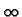, with G = 0 (Dirichlet) at x = 0.

 GX10(x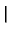x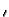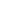) =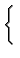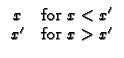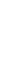X20 Semi-infinite body, 0 < x <, with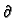G/x = 0 (Neumann) at x = 0. Note that this geometry requires a pseudo GF, denoted H. The temperature solution found from a pseudo GF requires that the total volumetric heating sums to zero and the spatial average temperature in the body must be supplied as a known condition.

 HX20(xx) =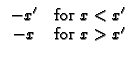X30 Semi-infinite body, 0 < x <, with - kG/x + hG = 0 (convection) at x = 0.

 GX30(xx) =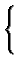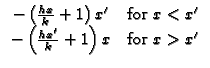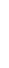Kevin D. Cole
2002-12-31